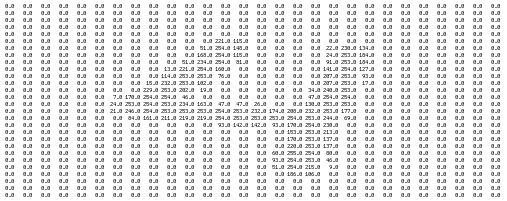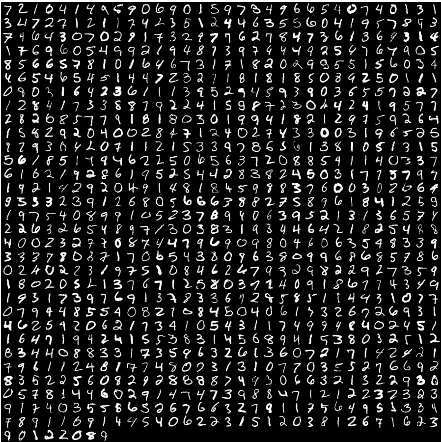29 February 2008

This post will show how to replicate the Matlab montage function using Python. The Data Wrangling blog seems to be getting search traffic from people learning python and looking for machine learning code, so I'm adding a few basic code snippets that you might find useful. Later posts will include Python examples that use the montage function to visualize pattern recognition and collaborative filtering algorithms.

In the past, I used Matlab for prototyping, but over the last few years I have switched to a combination of numpy, scipy, matplotlib, and ipython. When combined with the appropriate libraries, Python can have better numerical performance than Matlab or Octave, nearly identical functionality, and the additional flexibility of Python when you need to munge some text or expose your algorithm as a web service.

Anyway, lets get to the problem at hand... replicating the montage function. For this example, I dug up some data from a Sebastian Seung course on neural networks I took in 2005. The matfiles we used are now on Open Courseware. I think these are cropped versions of images from the MNIST database of handwritten digits (more image datasets here).

The raw dataset is stored in an array, where each row vector is a flattened version of a digitized grayscale image. If you select one vector, reshape it into a square array, and display it as an intensity plot, you get something like this:In grayscale:To display a montage of all the images (sometimes called a contact sheet), we will build a composite array where each submatrix is one of these reshaped rows. We also want to lay out the submatrices so that the result is roughly square, and all the empty elements are filled in with a default value. The end result looks like this:This functionality is built into the Matlab Image Processing Toolbox, but seems to be missing in matplotlib. Web searches turn up some similar code that uses the Python Imaging Library or examples based on Gimp, but they operate on image files instead of numpy matrices. I wanted something that closely replicated Matlab's montage() functionality which could be called interactively with matplotlib. Some additional digging turned up a nice octave script (in Finnish), which basically turns this post into a example of translating octave/matlab syntax into python.

Here is the Python equivalent of the montage function, montage.py:

``````import sys
import os
import time

from numpy import array,flipud,shape,zeros,rot90,ceil,floor,sqrt
from scipy import io,reshape,size
import pylab

def montage(X, colormap=pylab.cm.gist_gray):
m, n, count = shape(X)
mm = int(ceil(sqrt(count)))
nn = mm
M = zeros((mm * m, nn * n))

image_id = 0
for j in range(mm):
for k in range(nn):
if image_id >= count:
break
sliceM, sliceN = j * m, k * n
M[sliceN:sliceN + n, sliceM:sliceM + m] = X[:, :, image_id]
image_id += 1

pylab.imshow(flipud(rot90(M)), cmap=colormap)
pylab.axis('off')
return M``````

After reading in the Matlab files using Scipy's io.loadmat, the main method generates some example images using montage:

``````def main():
# This example loads greyscale face data which has been cropped into
# square matrices of length L.  The raw matlab data has one column
# for each face, which has been reshaped into a vector.
faces = faces_workspace['faces']

# This example creates a similar montage of handwritten digits from a
# sample of the the MNIST database
digits = digits_workspace['test']

for j, D in enumerate([faces, digits]):

try:
array_count = shape(D)
L = int(sqrt(shape(D)))
X = zeros((L, L, array_count))

for i in range(array_count):
X[:,:,i]= reshape(D[:,i], (L, L))

pylab.figure(j)
montage(X)

except MemoryError, detail:
print "MemoryError: ", detail

pylab.show()

if __name__ == '__main__':
main()``````

Here's the result when run on a similar dataset of grayscale faces:The montage code along with both data files can be downloaded here: montage.zip

To run the code, you will need to install numpy, scipy, and matplotlib if you don't already have them:

Fedora:

``sudo yum -y install scipy python-matplotlib``

Mac Ports:

``sudo port install py-scipy py-matplotlib``

Windows:

If you are a Matlab/Windows user thinking of switching to Python, then I would recommend starting with the latest release of the Enthought Python Distribution (nice one-click Windows install including numpy, scipy, etc). Travis Vaught just sent this announcement out to the Numpy mailing list:

For those of you unfamiliar with EPD, it's a "kitchen-sink-included" distribution of Python with over 60 additional tools and libraries. It's bundled into a nice MSI installer on Windows and includes NumPy, SciPy, IPython, 2D and 3D visualization, database adapters and a lot of other tools right out of the box ... EPD is compelling because it solves a lingering packaging and distribution problem, but also because of the libraries which it includes.

Some other good starting points for potential Matlab converts learning Python: